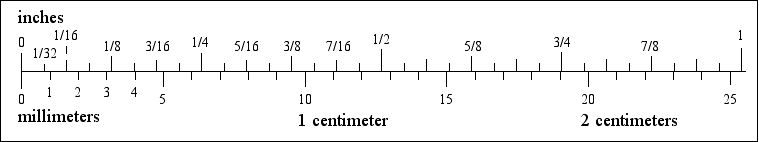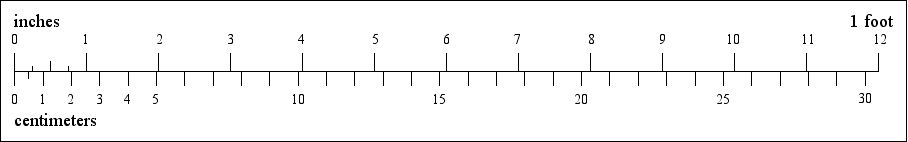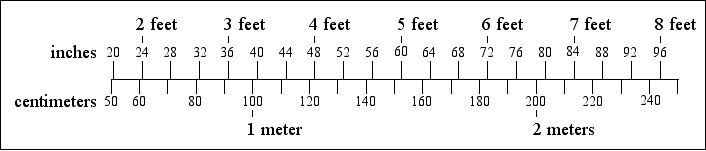# US and Metric Weights and Measures Compared

## Length 1

### inches to millimeters (mm)1 inch = 25.4 millimeter = 2.54 centimeter 1 millimeter = 0.04 inch 1 centimeter = 10 millimeter

### inches to centimeters (cm)1 inch = 2.54 cm 1 cm = 0.39 inch 12 inches = 1 foot = 30.48 centimeter

### inches and feet to centimeters and meters100 centimeters = 1 meter = 39.37 inches = 3.281 feetLength inches to millimeters and inches to centimeters feet to meters and yards to meters miles to kilometers fluid ounces to milliliters, cups to liters and gallons to liters ounces to grams and pounds to kilograms Fahrenheit to Celsius

Marco Schuffelen - email